# SSB Demodulation and Envelope detection

\$ 200,00

Stokta yok

Kategoriler:

## Açıklama

1) SSB  Demodulation:  Consider  the  message  waveform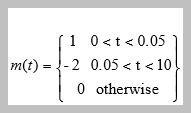and  the   SSB  modulated  signal mSSB-AM (T).You  can  use  here  whatever  SSB  method  you  like  to   use,  such  as  phase  method  or  Hilbert  transform  method.    First  obtain mSSB-AM (T) and demodulate  it  to  get mDEMOD (T).

Now  compare mDEMOD(T) and m(t) and illustrate the effect  of  local  oscillator  phase  errors,Ø, and mDEMOD(T).

show the distortions for Ø=Π/20 ,Ø=Π/6 and Ø=Π/2 ,by plotting the frequency spectra and waveforms of mDEMOD

(T)and m(t) on  separate  graphs.

2) Envelope  detection:  Demodulate  the  signal  s(t)=A⌈1+km(t)⌉cos(2Πƒct) ,  where  fc  =250  Hz,  and  k  =  0.85.    Plot  the  modulated  signal,  the  message  and  its  demodulated   version.  Try  filters  with  bandwidth  of  300  Hz  and  100  Hz.

3) Frequency  modulation:  Let  m(t)  modulate  a  carrier cos(2ΠƒT) where fc =200  Hz  and   frequency  deviation k=50  in  the  expression s(t)=Acos(2Πfct+2Π k∫m(s)ds. plot the  waveforms  and  spectra  of  the  message  signal  and  the  modulated  signal

4) Frequency  modulation:  LetThe  message  m(t)  modulates  a  carrier cos (2Πfct) where fc=250 Hz freqeuncy deviation kf=100 plot the  modulated  signal  in  the  time  and  frequency  domain.  Also  plot  the  waveforms  of  the   modulating  signal  and  its  demodulated  version  and  compare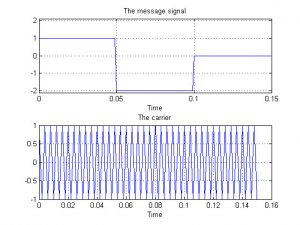MATLAB Handle Graphics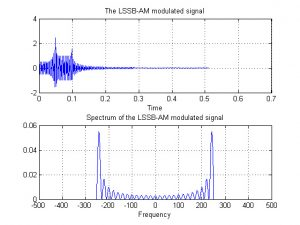MATLAB Handle Graphics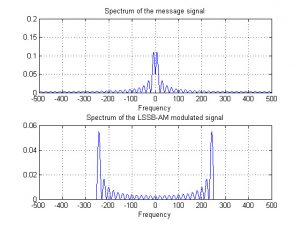MATLAB Handle Graphics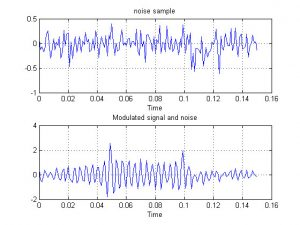MATLAB Handle Graphics2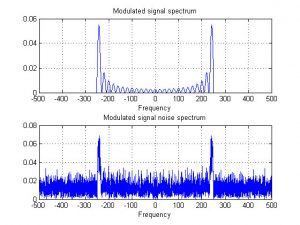MATLAB Handle Graphics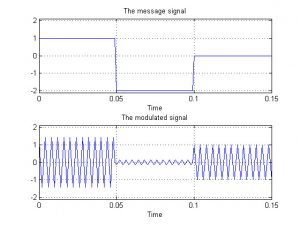MATLAB Handle Graphics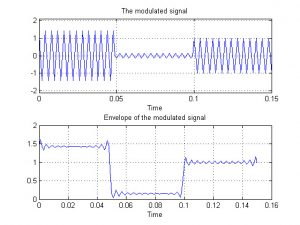MATLAB Handle GraphicsMATLAB Handle Graphics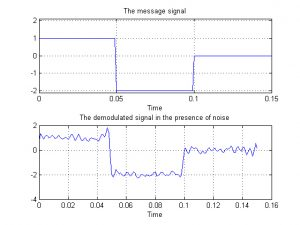MATLAB Handle Graphics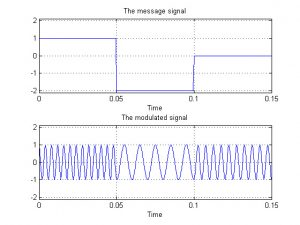MATLAB Handle Graphics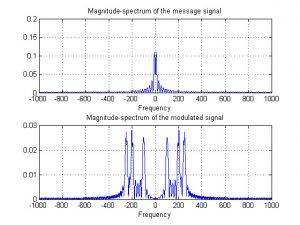MATLAB Handle Graphics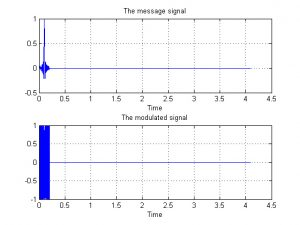MATLAB Handle Graphics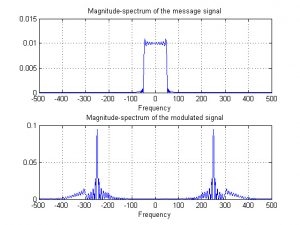MATLAB Handle Graphics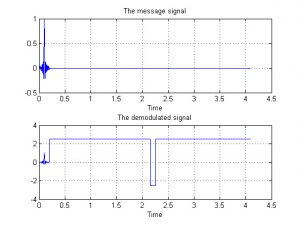MATLAB Handle Graphics